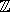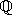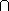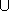## CGAL::Nef_polyhedron_S2<Traits>

### Definition

An instance of data type Nef_polyhedron_S2<Traits> is a subset of the sphere S2 that is the result of forming complements and intersections starting from a finite set H of halfspaces bounded by a plane containing the origin. Halfspaces correspond to hemispheres of S2 and are therefore modeled by oriented great circles of type Sphere_circle. Nef_polyhedron_S2 is closed under all binary set operations intersection, union, difference, complement and under the topological operations boundary, closure, and interior.

#include <CGAL/Nef_polyhedron_S2.h>

### Parameters

 template < class Nef_polyhedronTraits_S2, class Nef_polyhedronItems_S2 = CGAL::SM_items, class Nef_polyhedronMarks = bool class Nef_polyhedron_S2;

The first parameter requires one of the following exact kernels: Homogeneous, Simple_homogeneous parametrized with Gmpz, leda_integer or any other number type modeling, or Cartesian, Simple_cartesian parametrized with Gmpq, leda_rational,Quotient<Gmpz> or any other number type modeling.

The second parameter and the third parameter are for future considerations. Neither Nef_polyhedronItems_S2 nor Nef_polyhedronMarks is specifed, yet. Do not use other than the default types for these two template parameters.

### Types

 Nef_polyhedron_S2::Sphere_point points in the sphere surface. Nef_polyhedron_S2::Sphere_segment segments in the sphere surface. Nef_polyhedron_S2::Sphere_circle oriented great circles modeling spatial half-spaces. Nef_polyhedron_S2::SVertex_const_handle non-mutable handle to svertex. Nef_polyhedron_S2::SHalfedge_const_handle non-mutable handle to shalfedge. Nef_polyhedron_S2::SHalfloop_const_handle non-mutable handle to shalfloop. Nef_polyhedron_S2::SFace_const_handle non-mutable handle to sface. Nef_polyhedron_S2::SVertex_const_iterator non-mutable iterator over all svertices. Nef_polyhedron_S2::SHalfedge_const_iterator non-mutable iterator over all shalfedges. Nef_polyhedron_S2::SHalfloop_const_iterator non-mutable iterator over all shalfloops. Nef_polyhedron_S2::SFace_const_iterator non-mutable iterator over all sfaces. Nef_polyhedron_S2::SHalfedge_around_svertex_const_circulator circulating the adjacency list of an svertex v. Nef_polyhedron_S2::SHalfedge_around_sface_const_circulator circulating the sface cycle of an sface f. Nef_polyhedron_S2::SFace_cycle_const_iterator iterating all sface cycles of an sface f. The iterator has method bool is_svertex(), bool is_shalfedge(), bool is_shalfloop(), and can be converted to the corresponding handles SVertex_const_handle, SHalfedge_const_handle, or SHalfloop_const_handle. Nef_polyhedron_S2::Mark attributes of objects (vertices, edges, faces). Nef_polyhedron_S2::size_type size type enum Boundary { EXCLUDED , INCLUDED }; construction selection. enum Content { EMPTY , COMPLETE }; construction selection.

### Creation

Nef_polyhedron_S2<Traits> N ( Content sphere = EMPTY);
creates an instance N of type Nef_polyhedron_S2<K> and initializes it to the empty set if sphere == EMPTY and to the whole sphere if sphere == COMPLETE.

Nef_polyhedron_S2<Traits> N ( Sphere_circle c, Boundary circle = INCLUDED);
creates a Nef polyhedron N containing the half-sphere left of c including c if circle==INCLUDED, excluding c if circle==EXCLUDED.

template <class Forward_iterator>
 Nef_polyhedron_S2 N ( Forward_iterator first, Forward_iterator beyond, Boundary b = INCLUDED);
creates a Nef polyhedron N from the set of sphere segments in the iterator range [first,beyond). If the set of sphere segments is a simple polygon that separates the sphere surface into two regions, then the polygonal region that is left of the segment *first is selected. The polygonal region includes its boundary if b = INCLUDED and excludes the boundary otherwise. Forward_iterator has to be an iterator with value type Sphere_segment.

### Operations

 void N.clear ( Content plane = EMPTY) makes N the empty set if plane == EMPTY and the full plane if plane == COMPLETE. bool N.is_empty () returns true if N is empty, false otherwise. bool N.is_sphere () returns true if N is the whole sphere, false otherwise.

### Constructive Operations

 Nef_polyhedron_S2 N.complement () returns the complement of N in the plane. Nef_polyhedron_S2 N.interior () returns the interior of N. Nef_polyhedron_S2 N.closure () returns the closure of N. Nef_polyhedron_S2 N.boundary () returns the boundary of N. Nef_polyhedron_S2 N.regularization () returns the regularized polyhedron (closure of interior). Nef_polyhedron_S2 N.intersection ( Nef_polyhedron_S2 N1) returns NN1. Nef_polyhedron_S2 N.join ( Nef_polyhedron_S2 N1) returns NN1. Nef_polyhedron_S2 N.difference ( Nef_polyhedron_S2 N1) returns N - N1. Nef_polyhedron_S2 N.symmetric_difference ( Nef_polyhedron_S2 N1) returns the symmectric difference N - TT - N.

Additionally there are operators *,+,-,^,! which implement the binary operations intersection, union, difference, symmetric difference, and the unary operation complement respectively. There are also the corresponding modification operations *=,+=,-=,^=.

There are also comparison operations like <,<=,>,>=,==,!= which implement the relations subset, subset or equal, superset, superset or equal, equality, inequality, respectively.

### Statistics and Integrity

Size_type N.number_of_svertices ()
returns the number of svertices.
Size_type N.number_of_shalfedges ()
returns the number of shalfedges.

Size_type N.number_of_sedges ()
returns the number of sedges.
Size_type N.number_of_shalfloops ()
returns the number of shalfloops.
Size_type N.number_of_sloops ()
returns the number of sloops.
Size_type N.number_of_sfaces ()
returns the number of sfaces.
Size_type N.number_of_sface_cycles ()
returns the number of sface cycles.
Size_type N.number_of_connected_components ()
calculates the number of connected components of P.
void N.print_statistics ( std::ostream& os = std::cout)
print the statistics of P: the number of vertices, edges, and faces.
void
 N.check_integrity_and_topological_planarity ( bool faces=true)
checks the link structure and the genus of P.

### Exploration - Point location - Ray shooting

As Nef polyhedra are the result of forming complements and intersections starting from a set H of half-spaces that are defined by oriented lines in the plane, they can be represented by an attributed plane map M = (V,E,F). For topological queries within M the following types and operations allow exploration access to this structure.

### Types

 Nef_polyhedron_S2::Object_handle a generic handle to an object of the underlying plane map. The kind of object (vertex, halfedge, face) can be determined and the object can be assigned to a corresponding handle by the three functions: bool assign(Vertex_const_handle& h, Object_handle) bool assign(Halfedge_const_handle& h, Object_handle) bool assign(Face_const_handle& h, Object_handle) where each function returns true iff the assignment to h was done.

### Operations

bool N.contains ( Object_handle h)
returns true iff the object h is contained in the set represented by N.

bool N.contained_in_boundary ( Object_handle h)
returns true iff the object h is contained in the 1-skeleton of N.

Object_handle N.locate ( Sphere_point p)
returns a generic handle h to an object (face, halfedge, vertex) of the underlying plane map that contains the point p in its relative interior. The point p is contained in the set represented by N if N.contains(h) is true. The location mode flag m allows one to choose between different point location strategies.

Object_handle N.ray_shoot ( Sphere_point p, Sphere_direction d)
returns a handle h with N.contains(h) that can be converted to a Vertex_/Halfedge_/Face_const_handle as described above. The object returned is intersected by the ray starting in p with direction d and has minimal distance to p. The operation returns the null handle NULL if the ray shoot along d does not hit any object h of N with N.contains(h).

Object_handle
 N.ray_shoot_to_boundary ( Sphere_point p, Sphere_direction d)
returns a handle h that can be converted to a Vertex_/Halfedge_const_handle as described above. The object returned is part of the 1-skeleton of N, intersected by the ray starting in p with direction d and has minimal distance to p. The operation returns the null handle NULL if the ray shoot along d does not hit any 1-skeleton object h of N. The location mode flag m allows one to choose between different point location strategies.

### Iteration

 bool N.has_shalfloop () returns true iff there is a shalfloop. SHalfloop_const_handle N.shalfloop () returns access to the sloop.

The list of all objects can be accessed via iterator ranges. For comfortable iteration we also provide iterations macros. The iterator range access operations are of the following kind:
SVertex_iterator svertices_begin()/svertices_end()
SHalfedge_iterator shalfedges_begin()/shalfedges_end()
SHalfloop_iterator shalfloops_begin()/shalfloops_end()
SFace_iterator sfaces_begin()/sfaces_end()

The macros are then CGAL_forall_svertices(v,M), CGAL_forall_shalfedges(e,M), CGAL_forall_sfaces(f,M), CGAL_forall_sface_cycles_of(fc,F) where M is a sphere map and F is a sface.

### Input and Output

A Nef polyhedron N can be visualized in an open GL window. The output operator is defined in the file CGAL/IO/Nef_-poly-hedron_2_-Win-dow_-stream.h.

### Implementation

Nef polyhedra are implemented on top of a halfedge data structure and use linear space in the number of vertices, edges and facets. Operations like empty take constant time. The operations clear, complement, interior, closure, boundary, regularization, input and output take linear time. All binary set operations and comparison operations take time O(n logn) where n is the size of the output plus the size of the input.

The point location and ray shooting operations are implemented in the naive way. The operations run in linear query time without any preprocessing.

### Example

Nef polyhedra are parameterized by a standard CGAL kernel. The example computes the intersection of two Nef polyhedra N1 and N2.

```// examples/Nef_S2/simple.C

#include <CGAL/Gmpz.h>
#include <CGAL/Homogeneous.h>
#include <CGAL/Nef_polyhedron_S2.h>

typedef CGAL::Gmpz RT;
typedef CGAL::Homogeneous<RT> Kernel;
typedef CGAL::Nef_polyhedron_S2<Kernel> Nef_polyhedron;
typedef Nef_polyhedron::Sphere_circle Sphere_circle;

int main()
{
Nef_polyhedron N1(Sphere_circle(1,0,0));
Nef_polyhedron N2(Sphere_circle(0,1,0), Nef_polyhedron::EXCLUDED);
Nef_polyhedron N3 = N1 * N2;
return 0;
}
```